#Function Repository Resource:

# SimplexMeasure

Get the measure of a simplex or simplicial complex

Contributed by: Richard Hennigan (Wolfram Research)
 ResourceFunction["SimplexMeasure"][simplex] gives the measure of simplex. ResourceFunction["SimplexMeasure"][{simplex1,simplex2,…}] gives the measure of the simplicial complex containing simplex1,simplex2,…. ResourceFunction["SimplexMeasure"][complex,d] gives the d-dimensional measure of complex.

## Details and Options

A simplex can be specified by any of the following:
 Point[v] a point Line[{v1,v2}] a line segment Triangle[{v1,v2,v3}] or Polygon[{v1,v2,v3}] a filled triangle Tetrahedron[{v1,v2,v3,v4}] a filled tetrahedron Simplex[{v1,v2,…,vn}] an n-1 dimensional simplex
A simplicial complex can be specified by any of the following:
 {simplex1,simplex2,…} a list of simplices {{v1,2,…,v1,n},{v2,2,…,v2,n},…} a list of lists of vertices MeshRegion[…] a mesh region BoundaryMeshRegion[…] a boundary mesh region
ResourceFunction["SimplexMeasure"] uses the Cayley-Menger determinant to compute the measure of simplicies of arbitrary dimension.

## Examples

### Basic Examples

Get the measure of a Simplex:

 In:=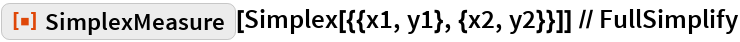Out=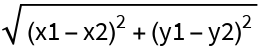Compare to Euclidean distance:

 In:=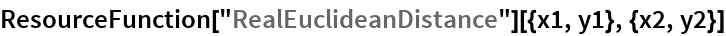Out=Get the measure of a Triangle:

 In:=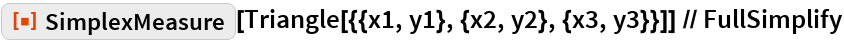Out=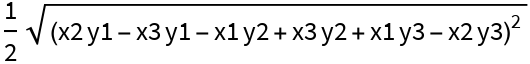Compare to Area:

 In:=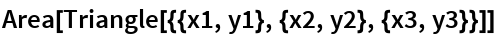Out=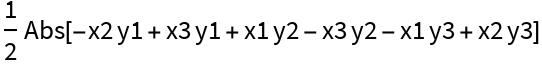Get the measure of a random 100-dimensional Simplex:

 In:=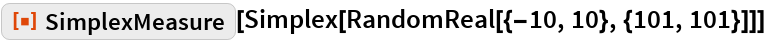Out=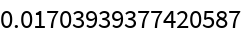Get the measure of a simplicial complex, represented as a list of simplices:

 In:=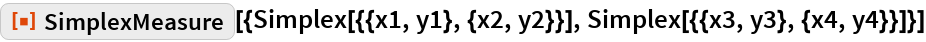Out=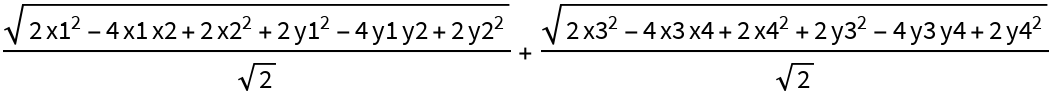Get the measure of a simplicial complex, represented by lists of vertices:

 In:=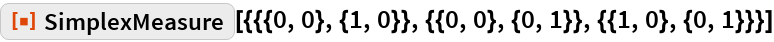Out=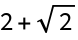Specify a dimension to measure:

 In:=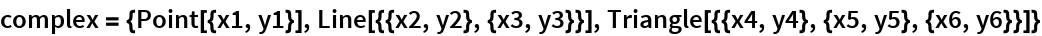Out=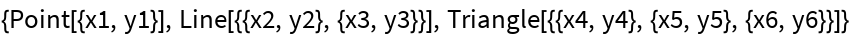In:=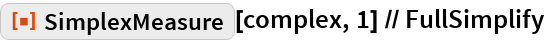Out=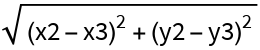In:=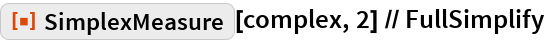Out=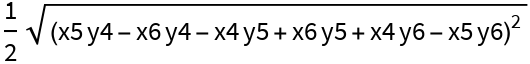Get the measure of a MeshRegion:

 In:=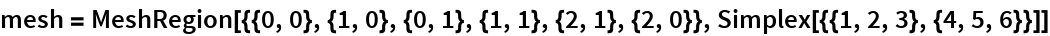Out=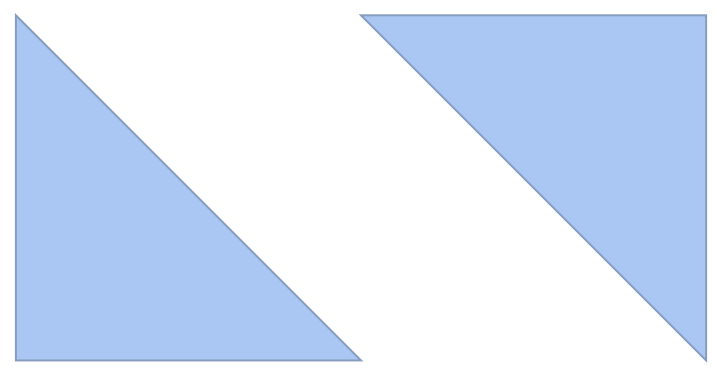In:=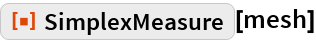Out=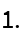### Properties and Relations

The measure for Point corresponds to counts:

 In:=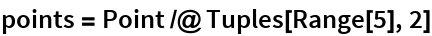Out=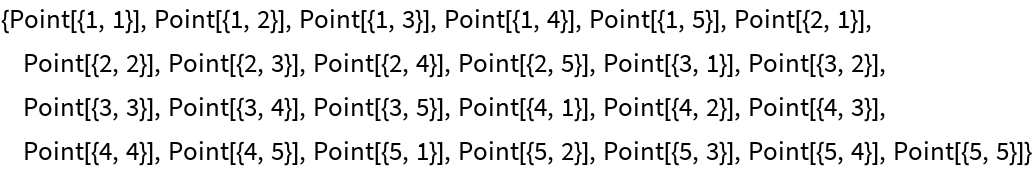In:=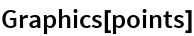Out=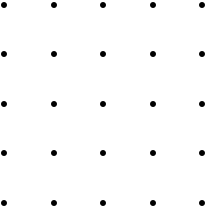In:=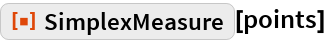Out=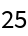For mesh regions, SimplexMeasure is equivalent to RegionMeasure:

 In:=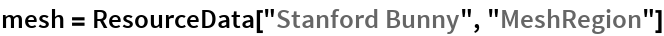Out=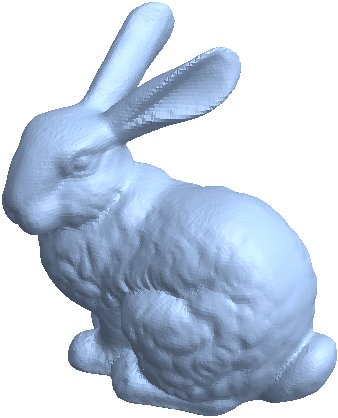In:=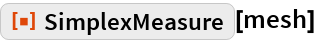Out=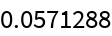In:=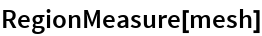Out=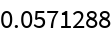SimplexMeasure works for arbitrary dimension:

 In:=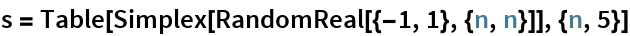Out=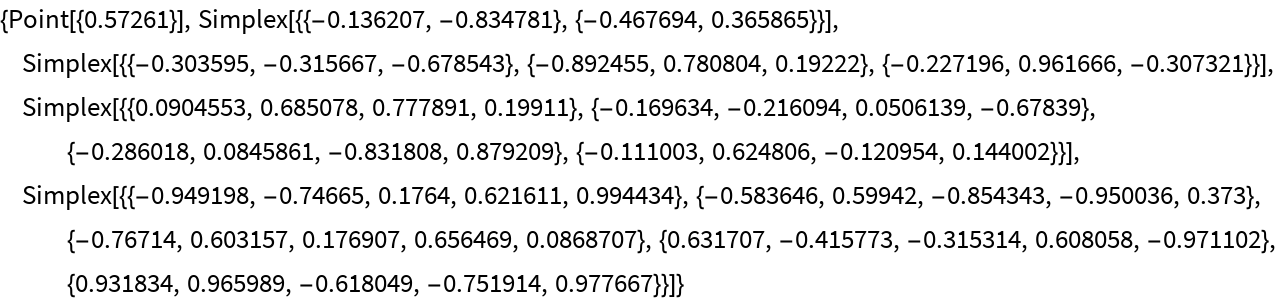In:=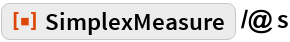Out=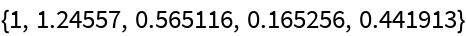Compare to RegionMeasure:

 In:=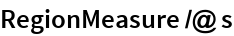Out=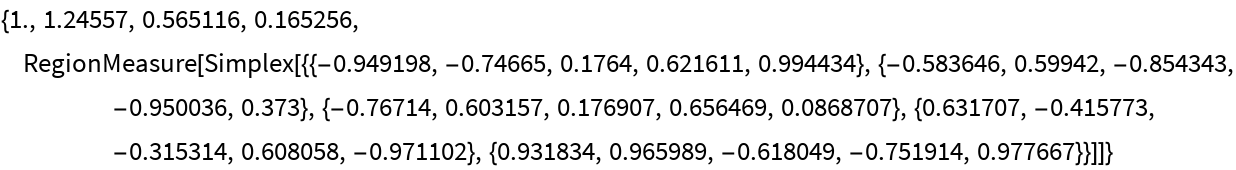SimplexMeasure performs best when given lists of vertices as an array:

 In:=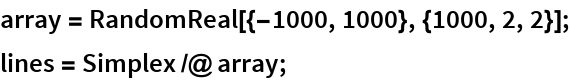In:=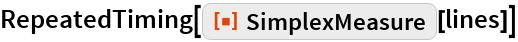Out=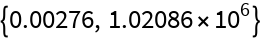In:=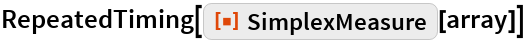Out=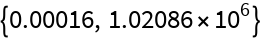Get the measure of the first 10 standard simplices:

 In:=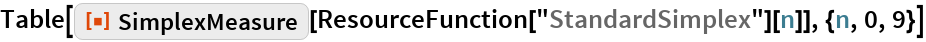Out=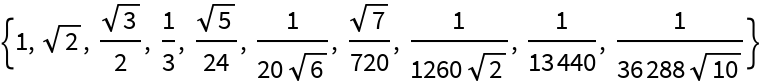In:=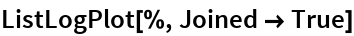Out=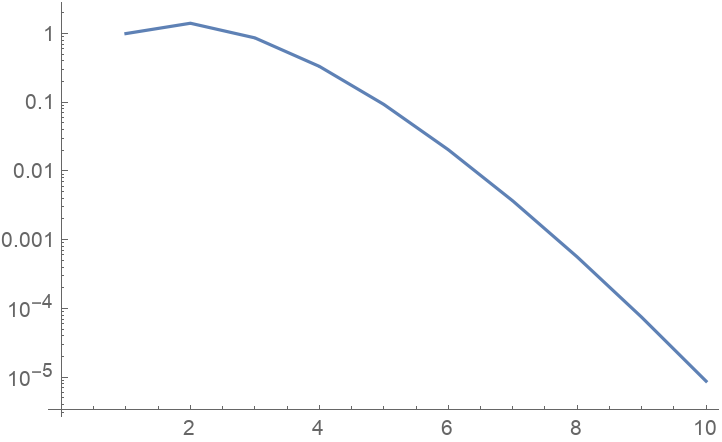Here’s the corresponding formula:

 In:=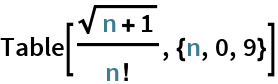Out=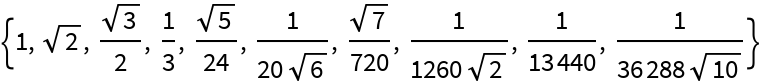SimplexMeasure uses more efficient methods for simplicial complexes below 6 dimensions:

 In:=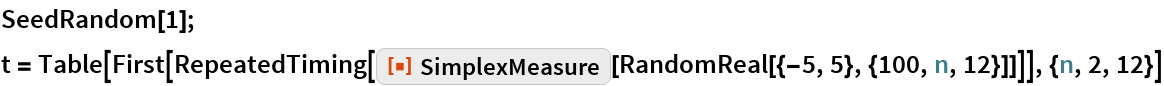Out=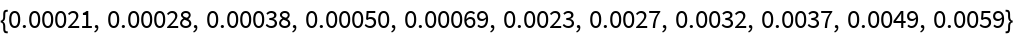In:=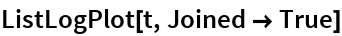Out=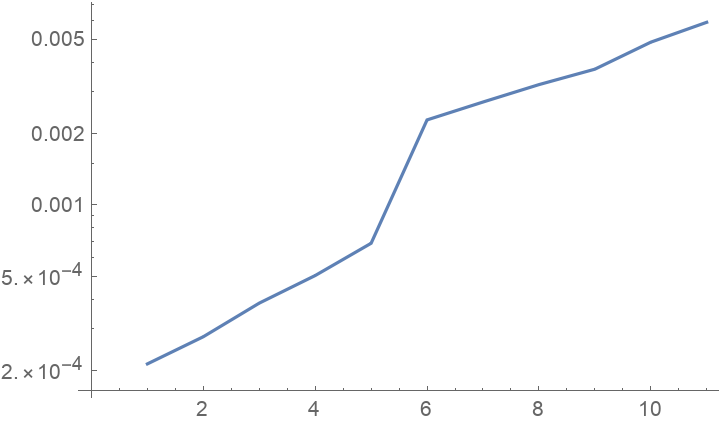Measure a simplex and its boundary:

 In:=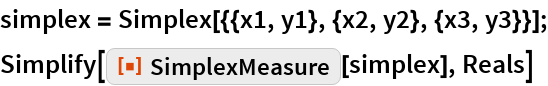Out=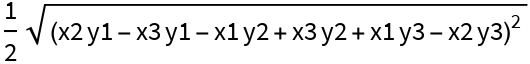In:=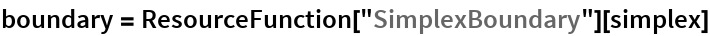Out=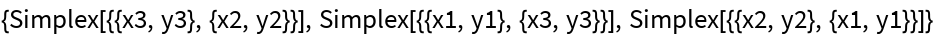In:=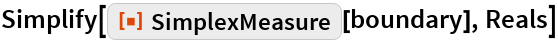Out=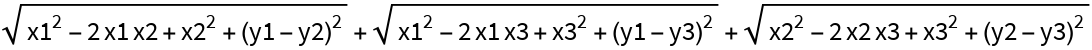### Possible Issues

SimplexMeasure is not supported for abstract simplices:

 In:=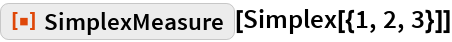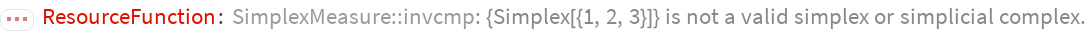Out=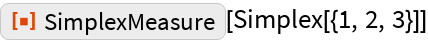In:=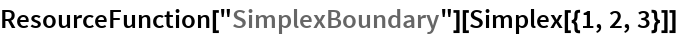Out=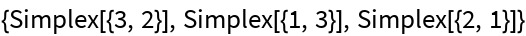In:=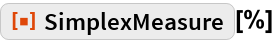Out=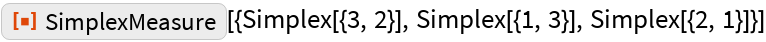## Requirements

Wolfram Language 11.3 (March 2018) or above